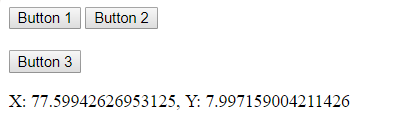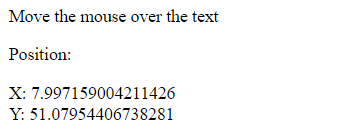# Retrieve the position (X,Y) of an element using HTML

The position of (X, Y) means the co-ordinate of an element at the top-left point in a document. X represent the horizontal position and Y represent the vertical position. Use element.getBoundingClientRect() property to get the position of an element.

Example 1:

 `` ` ` `<``html``> ` `    ``<``head``> ` `        ``<``title``> ` `            ``position of an element ` `        `` ` `         `  `        ``` `        ``<``script` `type``=``"text/javascript"``> ` `         `  `            ``function getPositionXY(element) { ` `                ``var rect = element.getBoundingClientRect(); ` `                ``document.getElementById('gfg').innerHTML =  ` `                ``'X: ' + rect.x + ', ' + 'Y: ' + rect.y ` `            ``} ` `        `` ` `    `` ` `     `  `    ``<``body``> ` `         `  `        ``` `        ``<``button` `id` `= ``'button1'` `onclick` `= ``"getPositionXY(this)"``> ` `            ``Button 1 ` `        `` ` `         `  `        ``<``button` `id` `= ``'button1'` `onclick` `= ``"getPositionXY(this)"``> ` `            ``Button 2 ` `        `` ` `         `  `        ``<``br``><``br``> ` `        ``<``button` `id` `= ``'button1'` `onclick` `= ``"getPositionXY(this)"``> ` `            ``Button 3 ` `        `` ` `         `  `        ``<``p` `id` `= ``'gfg'``> ` `     `  `    `` ` `                     `

Output:Example 2: In this example, move the pointer over the document to get the position of an element.

 `` ` ` `<``html``> ` `    ``<``head``> ` `        ``<``title``> ` `            ``position of an element ` `        `` ` `         `  `        ``` `        ``<``script` `type` `= ``"text/javascript"``> ` `            ``function getPositionXY(element) { ` `                ``var rect = element.getBoundingClientRect(); ` `                ``document.getElementById('text').innerHTML  ` `                ``= 'X: ' + rect.x + '<``br``>' + 'Y: ' + rect.y; ` `            ``} ` `        `` ` `    `` ` `     `  `    ``<``body``> ` `        ``<``p``>Move the mouse over the text ` `         `  `        ``<``div` `onmouseover` `= ``"getPositionXY(this)"``> ` `            ``Position: ` `            ``<``p` `id` `= ``'text'``> ` `        `` ` `     `  `    `` ` `                     `

Output:My Personal Notes arrow_drop_upCheck out this Author's contributed articles.

If you like GeeksforGeeks and would like to contribute, you can also write an article using contribute.geeksforgeeks.org or mail your article to contribute@geeksforgeeks.org. See your article appearing on the GeeksforGeeks main page and help other Geeks.

Please Improve this article if you find anything incorrect by clicking on the "Improve Article" button below.

Article Tags :

1

Please write to us at contribute@geeksforgeeks.org to report any issue with the above content.